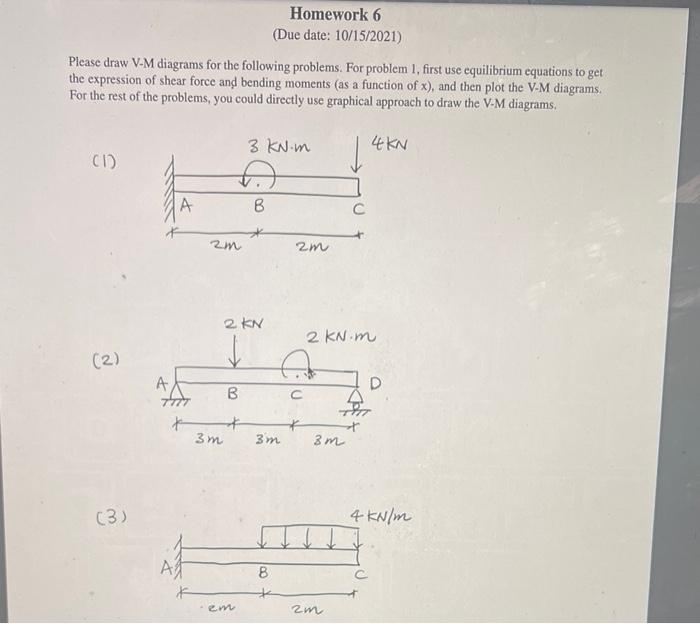# Please draw V-M diagrams for the following problems. For problem 1, first use equilibrium equations to get the expression of shear force and bending moments as a function of x), and then plot the V-M diagrams. For the rest of the problems, you could directly use graphical approach to draw the V-M

Question-AnswerCategory: Strength of MaterialsPlease draw V-M diagrams for the following problems. For problem 1, first use equilibrium equations to get the expression of shear force and bending moments as a function of x), and then plot the V-M diagrams. For the rest of the problems, you could directly use graphical approach to draw the V-M

Please draw V-M diagrams for the following problems. For problem 1, first use equilibrium equations to get the expression of shear force and bending moments as a function of x), and then plot the V-M diagrams. For the rest of the problems, you could directly use graphical approach to draw the V-M